# Logical Reasoning for MBA exam - Set 1

1)   In a coded message, SLOW MOVE, GET BACKWARDS, FIRE AWAY is written as VFMD ZMWE, BEN PCTLDCOXU, QHOE CDCI.
• Based on this coding scheme, spot the codes for following words
SALTY

a. UCFNI
b. UCFIN
c. UCINF
d. UCHIN

Explanation:

 Letter G E T A W Y F I R B C K D S M O V L Code B E N C D I Q H O P T L X U Z M W F
 Letter S A L T Y Code U C F N I

2)   Choose the number which is different from others in the group

a. 72 - 12
b. 48 – 24
c. 120 - 74
d. 96 - 84
 Answer  Explanation ANSWER: 120 - 74 Explanation: The HCF of all other numbers in the pair is 12.

1. Pepper Potts, Hulk, Ironman, Hawkeye, Thor, Loki and Black Widow are sitting in a circle.
2. Pepper Potts, Hulk, Ironman, Hawkeye, Loki and Black Widow are sitting at equal distances from each other.
3. Ironman is sitting two places right of Loki, who is sitting one place right of Hawkeye.
4. Pepper Potts forms an angle of 90° from Thor and an angle of 120° from Hulk.
5. Hulk is just opposite Black Widow and is sitting on the left of Thor.

Which of the following statements is not correct?

a. Loki is on the right of Hulk.
b. Hawkeye is sitting next to Ironman.
c. Thor is sitting adjacent to Loki.
d. All of the above
 Answer  Explanation ANSWER: All of the above Explanation: All of the above statements are not correct.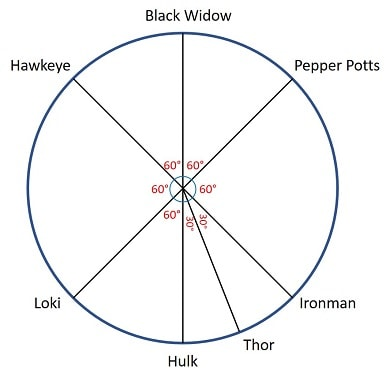4)   In the following question, various terms of an alphabet series are given with one or more missing terms as shown by (?). Choose the missing term out of the given alternatives.

XYA, XYC, XYB, XYD, XYC, ?

a. XYD
b. XYE
c. XYB
d. XYC
 Answer  Explanation ANSWER: XYE Explanation: In this series, XY remains constant while third letter changes in the pattern +2, -1, +2, -1 ….The next term should be XYE.

5)   In the question below is given a statement followed by three courses of action numbered I, II, III. You have to assume everything in the statement to be true and based on the information given in the statement, decide which of the suggested courses of action logically follow(s) for pursuing.

(A) If only I follows
(B) If only II follows
(C) If either I or II follows
(D) If neither I nor II follow
(E) If both I and II follow.

Statement - Number of persons affected by water-borne diseases is on a rise in India.

Courses of Action - I. The Legislative assembly should discuss the matter in the session.

II. The Government should spread adequate information regarding the pure drinking water to people.

III. Hospitals should be equipped with advanced facilities for the treatment.

a. Only I follows
b. Only II follows
c. Either I or II follows
d. Neither I nor II follows
e. Both II and III follow
 Answer  Explanation ANSWER: Both II and III follow Explanation: To tackle with any health problem, treatment and prevention are the two keys.Prevention includes creating awareness among people and treatment includes providing adequate medical facilities. So, II and III follow here.

6)   In the following question, a statement/group of statements is given followed by some conclusions. Without resolving anything yourself, choose the conclusion which logically follows from the statement(s).

Statement - All beggars are not poor.

a. Some beggars may not be poor.
b. If A is not rich, then A is not a beggar.
c. All those who are poor are beggars.
d. If A is rich, then A is not a beggar.
 Answer  Explanation ANSWER: Some beggars may not be poor. Explanation: No explanation is available for this question!

7)   Study the information given below carefully, and answer the question that follow:

On a stage, D, N, A and P are standing as described below facing North.
1) N is 2.5 m to the west of A.
2) K is 4 m to the right of A.
3) D is 6 m to the south of K.
4) P is 9 m to the north of D.

If a boy walks from N, meets A followed by K, D and then P, how many metres has he walked if he has travelled the straight distance all through?

a. 15 m
b. 18 m
c. 21.5 m
d. 22.5 m
 Answer  Explanation ANSWER: 21.5 m Explanation: Required distance = NA + AK + KD + DP = 2.5 + 4 + 6 + (6 + 3) = 21.5 m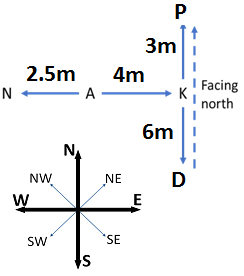6

8)   The angle between the minute hand and the hour hand of a clock when the time is 8.30, is

a. 65
b. 75
c. 85
d. 90
 Answer  Explanation ANSWER: 75 Explanation: At 8 o’ clock, the hour hand is at 240 degrees from the vertical.In 30 minutes,Hour hand = 240 + 30 x (0.5) = 240 + 15 = 255 (The hour hand moves at 0.5 dpm)Minute hand = 30 x (6) = 180 (The minute hand moves at 6 dpm)Difference or angle between the hands = 255 - 180 = 75 degrees.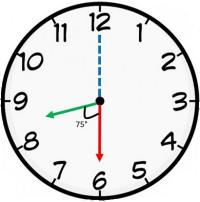9)   The question given below has a set of three or four statements. Each set of statements is further divided into three segments. Choose the alternative where the third segment in the statement can be logically deduced using both the preceding two, but not just from one of them.

Statement - A. All beggars are poor. All lions are poor. So, all lions are beggars.
B. All people are boxes. All foxes are people. So, all boxes are foxes.
C. All men can run. All women are men. So, all women can run.

a. A
b. B
c. C
d. None
 Answer  Explanation ANSWER: C Explanation: No explanation is available for this question!

10)   The question given below has a set of three or four statements. Each set of statements is further divided into three segments. Choose the alternative where the third segment in the statement can be logically deduced using both the preceding two, but not just from one of them.

Statement - I. All papers are books
II. All bags are books
III. Some purses are bags

Conclusion - I. Some papers are bags
II. Some books are papers
III. Some books are purses

a. Only I follows
b. Only II follows
c. Only III follows
d. Only I and II follows
e. Both II and III follow
 Answer  Explanation ANSWER: Both II and III follow Explanation: In this question, three possible diagrams following the given statements are possible. Before jumping to conclusion, one should verify whether the statements are true in all possible cases.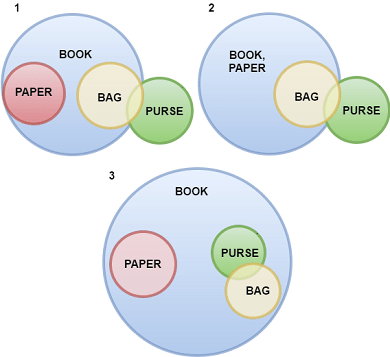11)   The question given below has a set of three or four statements. Each set of statements is further divided into three segments. Choose the alternative where the third segment in the statement can be logically deduced using both the preceding two, but not just from one of them.

Statement - I. Some guns are hammers.
II. Some hammers are bullets.
III. Some bullets are axes.

Conclusion - I. Some axes are hammers.
II. Some bullets are guns.
III. Some axes are guns.

a. Only I follows
b. Only II follows
c. Only III follows
d. Only I and II follows
e. None follows
 Answer  Explanation ANSWER: None follows Explanation: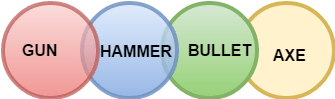12)   The question given below has a set of three or four statements. Each set of statements is further divided into three segments. Choose the alternative where the third segment in the statement can be logically deduced using both the preceding two, but not just from one of them.

Statement - I. All snakes are idiots.
II. Some idiots are people.
III. All people are soft.

Conclusion - I. Some idiots are not snakes.
II. Some people are snakes.
III. Some soft are idiots.

a. Only I follows
b. Only II follows
c. Only III follows
d. Only I and II follows
e. Both II and III follow
 Answer  Explanation ANSWER: Only III follows Explanation: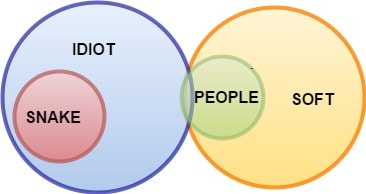13)   The question given below has a set of three or four statements. Each set of statements is further divided into three segments. Choose the alternative where the third segment in the statement can be logically deduced using both the preceding two, but not just from one of them.

Statement - I. Some buttons are rivers.
II. Some rivers are shirts.
III. All shirts are people.

Conclusion - I. Some people are rivers.
II. Some people are buttons.
III. Some shirts are buttons.

a. Only I follows
b. Only II follows
c. Only III follows
d. Only I and II follows
e. Both II and III follow
 Answer  Explanation ANSWER: Only I follows Explanation: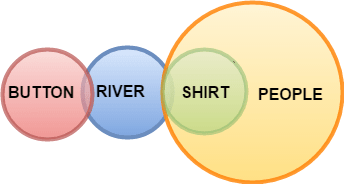14)   The question given below has a set of three or four statements. Each set of statements is further divided into three segments. Choose the alternative where the third segment in the statement can be logically deduced using both the preceding two, but not just from one of them.

Statement - I. All fruits are textbooks.
II. All pens are textbooks.
III. All textbooks are rains.

Conclusion - I. All fruits are rains.
II. All pens are rains.
III. Some rains are textbooks.

a. Only I follows
b. Only II follows
c. Only III follows
d. Only I and II follows
e. All follow
 Answer  Explanation ANSWER: All follow Explanation: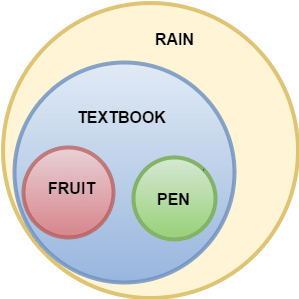15)   The following question consists of some Problem Figures followed by other figures marked 1, 2, 3, 4 called the Answer Figures.

Find out the correct answer figure that should come next in the sequence of problem figures.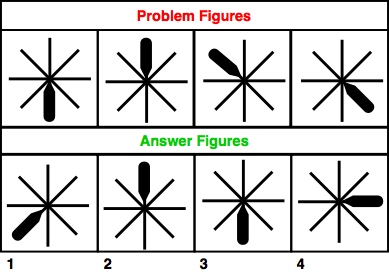a. 1
b. 2
c. 3
d. 4
 Answer  Explanation ANSWER: 4 Explanation: The bold bar is rotated through 180 degrees in first step and then through 45 degrees in anti-clockwise direction. The same step is repeated in a set order. The answer figure should be figure 4.

16)   The following question consists of some Problem Figures followed by other figures marked 1, 2, 3, 4 called the Answer Figures.

Find out the correct answer figure that should come next in the sequence of problem figures.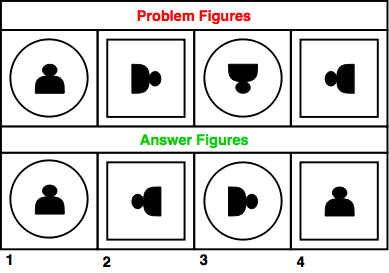a. 1
b. 2
c. 3
d. 4
 Answer  Explanation ANSWER: 1 Explanation: Circle and square outlines are alternatively changed and the figure inside is rotated through 90 degrees in clockwise direction. The answer figure should be figure 1.

17)   The following question consists of some Problem Figures followed by other figures marked 1, 2, 3, 4 called the Answer Figures.

Find out the correct answer figure that should come next in the sequence of problem figures.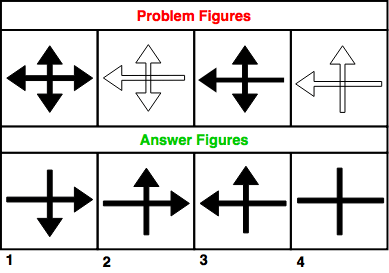a. 1
b. 2
c. 3
d. 4
 Answer  Explanation ANSWER: 3 Explanation: In the first step, triangle element is deleted and shallow figure is obtained.In the second step, shallow figure is replaced by filled one. This pattern is followed and repeated. Thus, the answer figure should be figure 3.

18)   The following question consists of some Problem Figures followed by other figures marked 1, 2, 3, 4 called the Answer Figures.

Find out the correct answer figure that should come next in the sequence of problem figures.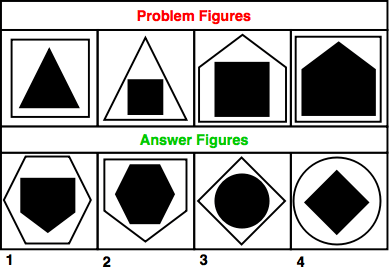a. 1
b. 2
c. 3
d. 4
 Answer  Explanation ANSWER: 1 Explanation: 3-sided figure is inside 4-sided and in the next step it is reversed.Similarly, 4-sided figure is inside 5-sided figure and vice versa.Thus, following the same order, the answer figure should be figure 1.

19)   The following question consists of some Problem Figures followed by other figures marked 1, 2, 3, 4 called the Answer Figures.

Find out the correct answer figure that should come next in the sequence of problem figures.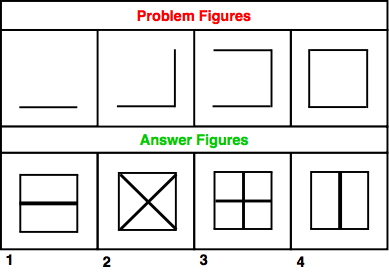a. 1
b. 2
c. 3
d. 4
 Answer  Explanation ANSWER: 1 Explanation: Vertical and horizontal lines are added in each step to obtain a complete figure. Thus, the answer figure should be figure 1.

20)   The following question consists of some Problem Figures followed by other figures marked 1, 2, 3, 4 called the Answer Figures.

Find out the correct answer figure that should come next in the sequence of problem figures.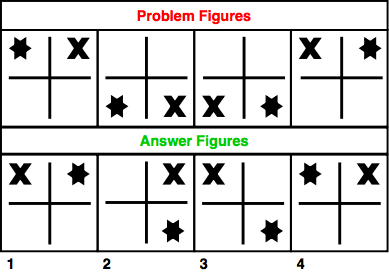a. 1
b. 2
c. 3
d. 4
 Answer  Explanation ANSWER: 4 Explanation: X moves in clockwise direction and star moves in anti-clockwise direction in a set order. Thus, the answer figure should be figure 4.